Next: Normal Modes Up: Coupled Oscillations Previous: Stability Equations

More Matrix Eigenvalue Theory

Equation (785) takes the form of a matrix eigenvalue equation: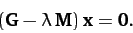(786)

Here,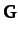and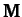are both real symmetric matrices, whereas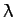is termed the eigenvalue, andthe associated eigenvector. The above matrix equation is essentially a set of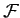homogeneous simultaneous algebraic equations for the components of. As is well-known, a necessary condition for such a set of equations to possess a non-trivial solution is that the determinant of the matrix must be zero: i.e.,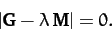(787)

The above formula reduces to anth-order polynomial equation for. Hence, we conclude that Equation (786) is satisfied byeigenvalues, andassociated eigenvectors.

We can easily demonstrate that the eigenvalues are all real. Suppose that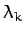and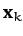are the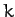th eigenvalue and eigenvector, respectively. Then we have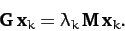(788)

Taking the transpose and complex conjugate of the above equation, and right multiplying by, we obtain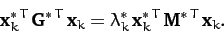(789)

Here,denotes a transpose, anda complex conjugate. However, sinceandare both real symmetric matrices, it follows that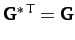and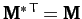. Hence,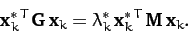(790)

Next, left multiplying Equation (788) by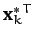, we obtain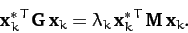(791)

Taking the difference between the above two expressions, we get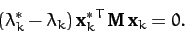(792)

Since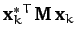is not generally zero, except in the trivial case whereis a null vector, we conclude that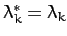for all. In other words, the eigenvalues are all real. It immediately follows that the eigenvectors can also be chosen to be all real.

Consider two distinct eigenvalues,and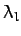, with the associated eigenvectorsand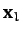, respectively. We have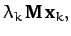(793)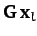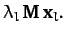(794)

Right multiplying the transpose of Equation (793) by, and left multiplying Equation (794) by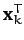, we obtain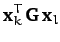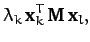(795)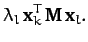(796)

Taking the difference between the above two expressions, we get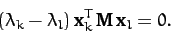(797)

Hence, we conclude that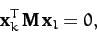(798)

provided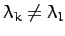. In other words, two eigenvectors corresponding to two different eigenvalues are orthogonal'' to one another (in the sense specified in the above equation). Moreover, it is easily demonstrated that different eigenvectors corresponding to the same eigenvalue can be chosen in such a manner that they are also orthogonal to one another--see Section 8.5. Thus, we conclude that all of the eigenvectors are mutually orthogonal. Since Equation (786) only specifies the directions, and not the lengths, of the eigenvectors, we are free to normalize our eigenvectors such that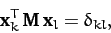(799)

where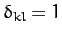when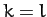, and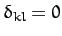otherwise. Note, finally, that since the, for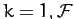, are mutually orthogonal, they are independent (i.e., one eigenvector cannot be expressed as a linear combination of the others), and completely span-dimensional vector space.Next: Normal Modes Up: Coupled Oscillations Previous: Stability Equations
Richard Fitzpatrick 2011-03-31